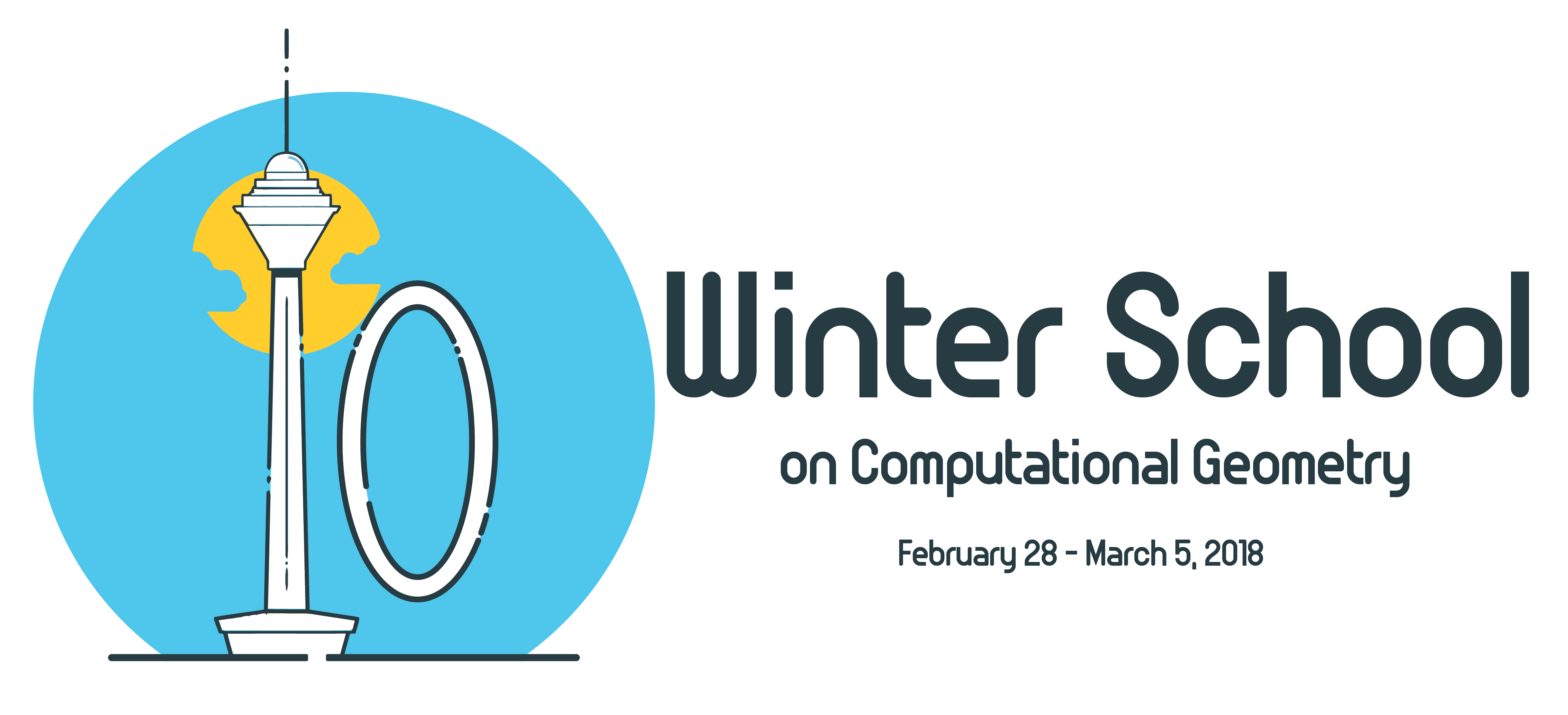# 10th Winter School on Computational Geometry

## 10th Winter School on Computational Geometry

#### Helmut Alt

COMPUTATIONAL ASPECTS OF PACKING PROBLEMS

#### Jean-Daniel Boissonnat

Delaunay triangulation of manifolds

• 1. Delaunay triangulations in Euclidean and Laguerre geometry
1.1. Euclidean case, Delaunay's theorem.
1.2. Weighted points. Affine diagrams. Union of balls.
1.3. Examples of affine diagrams.

• 2. Good Delaunay triangulations and meshes
2.1. Nets and combinatorial bounds. Delaunay refinement.
2.2. Thickness, protection, stability of Delaunay triangulations.
2.3. Perturbation algorithms. The Local Lovasz Lemma and heuristics.

• 3. Triangulation of topological spaces
3.1. Topological spaces, equivalences between topological spaces.
3.2. (Abstract) simplicial complexes, nerve theorem.
3.3. Geometric complexes and filtrations : Cech, Rips, alpha-complexes.
3.4. Data structures for simplicial complexes.

• 4. Reconstruction of submanifolds of Rd
4.1. Sets of positive reach.
4.2. Quality of approximation.
4.3. Distance functions and homotopic shape reconstruction.
4.4. The curse of dimensionality, the tangential Delaunay complex.

• 5. Delaunay triangulation of Riemannian manifolds
5.1. Anisotropic Delaunay triangulation.
5.2. Delaunay triangulation of Riemannian manifolds.
5.3. Statistical spaces. Fischer metric and Bregman divergence.
• Delaunay triangulations of manifolds
• Delaunay triangulation of manifolds 2 (Good triangulations and meshes)
• Delaunay triangulation of manifolds 3 (Triangulation of topological spaces)
• Delaunay triangulation of manifolds 4 (Shape reconstruction)
• Delaunay triangulation of manifolds 5

#### Carola Wenk

Algorithms for Map Construction and Comparison

• Map-Matching
Frechet distance
Frechet map-matching
HMM map-matching

• Map Construction I
Density-based methods
Frechet-based

• Map Construction II
Frechet-clustering
Bundle map-construction

• Map Comparison
Hausdorff distance
Path-based distances
Local persistent homology-based distance
Graph sampling-based distance
Local signatures

• Extensions
Handling directed edges, multiple lanes, turn restrictions
Map comparison for directed and weighted graphs